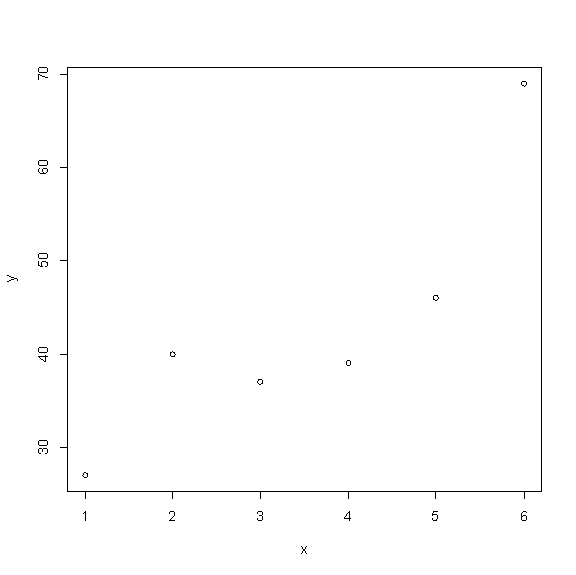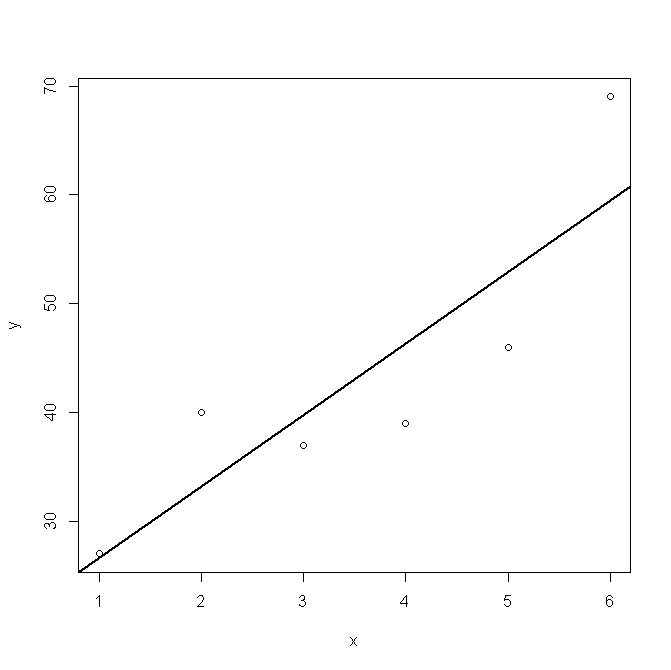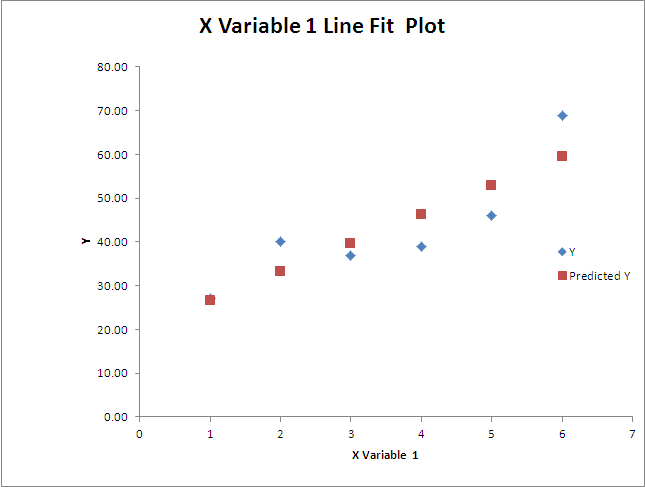25 Oct

## Linear Regression (R)

While doing linear regression using R, following error is obtained:

Error in int_abline(a = a, b = b, h = h, v = v, untf = untf, …) :
plot.new has not been called yet

This results in visually unable to see the line on the scatterplot. Though examining the summary of the model confirms that model has been built rightly and coefficients values can be obtained as well. Well this error is not an error rather it boils down to the fact that we need to use R differently. Following lines will make this clear.

Say, we want to forecast number of calls based on few months of data. The data is available as following:

1         27
2         40
3         37
4         39
5         46
6         69

Step 1 . Save the data in CSV format. (data.csv)

Step 3. Assign x and y variables from c. x <-  c\$Month and y <-  c\$Calls

`Step 4. Create the model. mod1 <- lm(y ~ x)`

Step 5. Scatterplot – plot (x, y, type = “p”)Above window will be shown.  Now closing above window and making the call `abline(mod1, lwd=2)` results in the error   “plot.new has not been called yet

The reason for above is since the scatter plot window is closed R could not find a place to draw the line. The solution lies in to click the button “Return focus to Console” and then call the function `abline(mod1, lwd=2)``on R Console`. The result is as following.Quite easy!!!! isn’t it?

Taking this further , the linear equation obtained is  Calls = 6.5 X Months + 20.00. As per this estimate 65,    72 and   78.5 calls are expected in next three months.

The same analysis can be done thrugh excel and the resulting model is as following.Plugin from the creators ofBrindes Personalizados :: More at PlulzWordpress Plugins